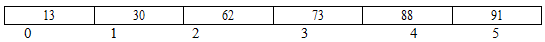## How to write binary search algorithm?, Data Structure & Algorithms

Assignment Help:

Q. Write down the binary search algorithm and trace to search element 91 in following given list:

13          30          62           73         81         88             91

What are the major limitations of Binary Search?

Ans.

13   30   62   73   88   91

lets us store the numbers in an arrayTo start with we take low = 0 and high = 5 hence mid = (low+high)/2 = (0+5) /2 = 2

since a[mid] = a = 62 ≠ 91 and low < high we go to next iteration as 62 < 91

∴we take low = mid+1 = 3 and high =

now we find mid =( 3+5)/2 = 4

∴a [mid] = a = 88.

88 is not equal to 91

again 88< 91

∴ we take low = mid +1 = 4 + 1 = 5 and high = 5

∴mid = (5+5)/2 = 5

∴ a[mid] = a = 91 which is the search element.

Limitation of Binary Search is given below: -

(i) The complexity of Binary search is O(log2 n)  the complexity is same irrespective of the  location of the element, even if it is not there in the array.

(ii) The algorithm makes an assumption that one has direct access to middle element in the list on a sub list. This means that the list must be stored in some type of array. Unfortunately inserting an element in an array requires element to be moved down the list and deleting an element from an array requires element to be moved up the list.

(iii) The list must be sorted.

#### Binary search, In a sorted list, Binary search is carried out by dividing t...

In a sorted list, Binary search is carried out by dividing the list into two parts depends on the comparison of the key. Since the search interval halves each time, the iteration o

#### Write an algorithm outputs number of books using psuedocode, A shop sells b...

A shop sells books, maps and magazines. Every item is identified by a unique 4 - digit code. All books have a code starting with a 1, all maps have a code which starts with a 2 and

#### Write a procedure that produces independent stack, Write a procedure (make-...

Write a procedure (make-stack) that produces independent stack objects, using a message-passing style, e.g. (define stack1 (make-stack))  (define stack2 (make-stack)) W

#### Add the special form let to the metacircular interpreter, (1)  (i) Add the ...

(1)  (i) Add the special form let to the metacircular interpreter Hint: remember let is just syntactic sugar for a lambda expression and so a rewrite to the lambda form is all t

#### HUFFMAN CODING, Ask question 1. Indicate whether each of the following prop...

Ask question 1. Indicate whether each of the following properties is true for every Huffman code. a. The codewords of the two least frequent symbols have the same length. b. The

#### Breadth-first search , 1. Apply the variant Breadth-First Search algorithm ...

1. Apply the variant Breadth-First Search algorithm as shown in Figure 2 to the attached graph. This variant is used for computing the shortest distance to each vertex from the sta

#### Algorithm, give me algorithm of simple interest

give me algorithm of simple interest

#### The two famous methods for traversing, The two famous methods for traversin...

The two famous methods for traversing are:- a) Depth first traversal b) Breadth first

#### Find strongly connected components - dfs, A striking application of DFS is ...

A striking application of DFS is determine a strongly connected component of a graph. Definition: For graph G = (V, E) , where V refer to the set of vertices and E refer to the